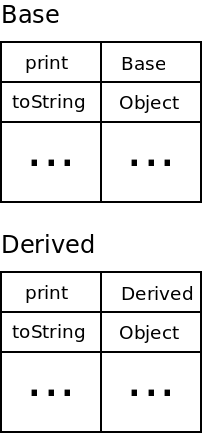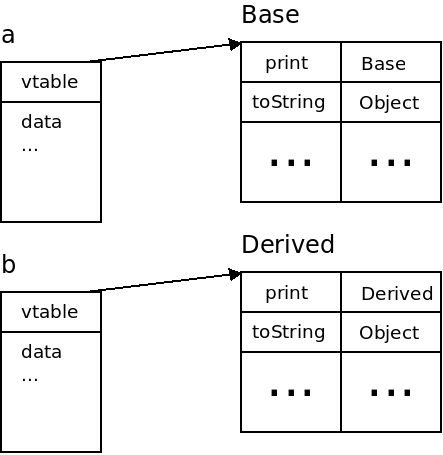# Implementing Functions

## Overview

Implementing functions involves a few different things:

• Passing parameters.
• Transferring control.
• Specifying a return value
• Transferring to the correct location.

Other language features such as overloading, recursion, nested functions and templates require the compiler to implement them specially.

## Calling Conventions

The way that functions are implemented can depend on the machine, language and compiler being used. The details of how functions are called, and parameters passed is called the calling convention.

C and C++ compilers on x86 typically uses the cdecl calling convention:

• All arguments are passed on the stack (in reverse order).
• Values are returned in the eax register.

The following C code:


int add(int x, int y) {
return x + y;
}

int main() {
}

Could be compiled into the following assembly (simplified somewhat):

movl   (%esp), %eax   ; read arg1
movl  4(%esp), %edx   ; read arg2
ret                   ; jump back

main:
subl  $8, %esp ; make room on the stack movl$7, 4(%esp)     ; push the 7
movl  \$12, (%esp)     ; push the 12
call  add             ; call the function


Other calling conventions specify that some arguments are passed via registers.

## Byte Code

Languages that compile to intermediate code (such as Java and C#) also use calling conventions. The following Java code:


public class Example {
public static int add(int x, int y) {
return x + y;
}

public static void main(String[] args) {
}
}


Compiles to the following Java byte code:


Code:
3:   ireturn

public static void main(java.lang.String[]);
Code:
0:   bipush  12
2:   bipush  7
7:   istore_1
8:   return


The JVM calling convention is that arguments are pushed onto the stack in order. The JVM also uses the stack for the return value (the add automatically pushes its result).

## Calls & Returns

When a function returns, it has to know exactly where to return to. This is accomplished by passing the function the return address when it is called.

The way this is done depends on the architecture and calling convention being used. In x86, this is done by pushing the return address onto the stack (some architectures use a register instead).

Each time a function is called, a stack frame is pushed onto the stack with parameters, locals and the return address. Each time one returns, its stack frame s popped.

## Recursion

Including recursion makes the call and return process slightly more complicated. Now, a function can have multiple stack frames at the same time.


int factorial(int x) {
if(x < 2) {
return 1;
} else {
return x * factorial(x - 1);
}
}

int main() {
factorial(6);
}


The stack for this code at the end of the recursion would look like this:Using a stack for parameters and the return address is natural for recursion. With a calling convention that uses registers, recursive functions must push them to the stack as well.

## Closures

Closures require care to implement correctly. Consider the following example closure:


# return a counter function
def counter(start, step):
i = start - step
def c():
nonlocal i
i += step
return i
return c

# create counter functions
counter1 = counter(1, 1)
counter2 = counter(100, -1)


The closure that is returned needs access to the local variables of the counter function, i and step. However, once the counter function returns its locals are normally removed from the stack.

There are multiple ways to get around this:

• Copy the outer functions stack frame into a closure data structure.
• Rewrite the code for the closure dynamically to contain the values it needs.
• Allocate all locals on the heap instead of the stack (and let a GC deal with them).

This difficulty is why closures are typically included in dynamic languages and not static ones.

## Name Mangling

Many languages provide overloading of functions and operators. Thus we can write the following code in C++:


int function() {
return 3;
}

int function(int x, char c) {
return x + c;
}

int function(string s, float* f) {
return s.length() + (int) *f;
}


However, assembly languages typically do not allow function overloading. To get around this problem, C++ compilers change function names to include their arguments. g++ compiles these functions as:


_Z8functionv

_Z8functionic

_Z8functionSsPf


The _Z is used for all functions by g++. The 8 gives the length of the actual name "function". Last is a code describing the types of parameters.

Name mangling schemes are different from compiler to compiler.

## Templates

When using templates in C++, the compiler generates one copy of the template function for each different version that is used. For example with the Max function:


template
type Max(type a, type b) {
a > b ? a : b;
}

int main() {
cout << "Max(3, 4) = " << Max(3, 4) << endl;
cout << "Max(v, c) = " << Max('v', 'c') << endl;
cout << "Max(2.5, 5.8) = " << Max(2.5, 5.8) << endl;

return 0;
}


The compiler creates three copies of this function, one that takes ints, chars and doubles.

These functions are compiled by g++ as:


_Z3MaxIiET_S0_S0_

_Z3MaxIcET_S0_S0_

_Z3MaxIdET_S0_S0_


This also allows for different implementations for each function. The machine code to compare ints and floats is different.

## Late Binding

The functions we have discussed so far have used static binding of function calls to function bodies. However, with polymorphism, we can also have dynamic, or late binding to functions:


class Base {
public void print() {
System.out.println("Base!");
}
}

class Derived extends Base {
public void print() {
System.out.println("Derived!");
}
}

public class Example {
public static void main(String[] args) {
Base a, b;

a = new Base();
b = new Derived();

a.print();
b.print();
}
}


Here the calls to print must use late binding. When generating code, the compiler cannot know for certain which function to match a call to "print" with.

This is handled with a virtual method table or vtable. This table matches function names to where they are defined. There is one vtable created for each class.Then each object that is created stores a pointer to the virtual table of the class it currently stores:Every time a function is called, the function's location is first looked up inside of the vtable.